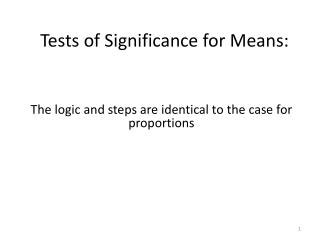Download PresentationTests of Significance for Means:

# Tests of Significance for Means: - PowerPoint PPT PresentationDownload Presentation## Tests of Significance for Means:

- - - - - - - - - - - - - - - - - - - - - - - - - - - E N D - - - - - - - - - - - - - - - - - - - - - - - - - - -
##### Presentation Transcript

1. Tests of Significance for Means: The logic and steps are identical to the case for proportions

2. Activity 1: Backpack weight

3. Activity 1: Watch Outs !! Remember: Hypotheses are always about (population) parameters, not statistics. The alternative hypothesis should always be formulated before collecting the sample data, and based on the research question. When calculating the p-value for a two-sided alternative, include the total area in both tails of the t-distribution and calculate this total area by doubling the area in the right tail. Always include ALL six steps of a significance test: 1. Identify and define the parameter 2. State the null and alternative hypothesis, preferably in words as well as symbols 3. Check the technical conditions 4. Calculate the test statistic 5. Report the p-value 6. Summarize your conclusion in context, using a test decision if a significance level is provided

4. Activity 2: Child television viewing To be completed at home (but not submitted)

5. Summary of the significance test procedure for means

6. Summary of the significance test procedure for means

7. Consequences of test decisions Suppose you conduct a significance test, and on its basis decide to reject the null hypothesis. If the null hypothesis is actually false, then you will have made a good (correct) decision. BUT, could you have made a wrong decision? That is, is it possible that despite the test decision, the null hypothesis is actually true? How could such an error occur?

8. Consequences of test decisions Suppose you conduct a significance test, and on its basis decide not to reject the null hypothesis. If the null hypothesis is actually true, then you will have made a good (correct) decision BUT, could you have made a wrong decision? That is, is it possible that despite the test decision, the null hypothesis is actually false? How could such an error occur?

9. Consequences of test decisions These two types of errors are so important in making statistical decisions on the basis of significances that they have been given their own special designation: Type I and Type II errors. See the animated activity in ActivitStatssection V.19.4 for a nice explanation of these types of errors.